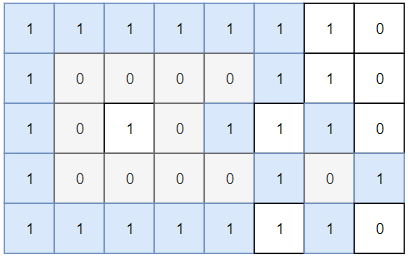Formatted question description: https://leetcode.ca/all/1254.html

# 1254. Number of Closed Islands (Medium)

Given a 2D grid consists of 0s (land) and 1s (water).  An island is a maximal 4-directionally connected group of 0s and a closed island is an island totally (all left, top, right, bottom) surrounded by 1s.

Return the number of closed islands.

Example 1:Input: grid = [[1,1,1,1,1,1,1,0],[1,0,0,0,0,1,1,0],[1,0,1,0,1,1,1,0],[1,0,0,0,0,1,0,1],[1,1,1,1,1,1,1,0]]
Output: 2
Explanation:
Islands in gray are closed because they are completely surrounded by water (group of 1s).

Example 2:Input: grid = [[0,0,1,0,0],[0,1,0,1,0],[0,1,1,1,0]]
Output: 1


Example 3:

Input: grid = [[1,1,1,1,1,1,1],
[1,0,0,0,0,0,1],
[1,0,1,1,1,0,1],
[1,0,1,0,1,0,1],
[1,0,1,1,1,0,1],
[1,0,0,0,0,0,1],
[1,1,1,1,1,1,1]]
Output: 2


Constraints:

• 1 <= grid.length, grid.length <= 100
• 0 <= grid[i][j] <=1

Related Topics: Depth-first Search

## Solution 1. DFS

// OJ: https://leetcode.com/problems/number-of-closed-islands/
// Time: O(MN)
// Space: O(1)
class Solution {
int dirs = { {0,1},{0,-1},{-1,0},{1,0} }, M, N;
bool dfs(vector<vector<int>> &G, int x, int y, int id) {
G[x][y] = id;
bool ans = true;
for (auto &[dx, dy] : dirs) {
int a = x + dx, b = y + dy;
bool oob = a < 0 || b < 0 || a >= M || b >= N;
ans = ans && !oob;
if (oob || G[a][b] != 0) continue;
ans = dfs(G, a, b, id) && ans;
}
return ans;
}
public:
int closedIsland(vector<vector<int>>& G) {
int id = 2, ans = 0;
M = G.size(), N = G.size();
for (int i = 0; i < M; ++i) {
for (int j = 0; j < N; ++j) {
if (G[i][j] != 0) continue;
if (dfs(G, i, j, id++)) ++ans;
}
}
return ans;
}
};


Java

• class Solution {
final int WATER = -1;
final int WHITE = 0;
final int GRAY = 1;
final int BLACK = 2;

public int closedIsland(int[][] grid) {
if (grid == null || grid.length == 0 || grid.length == 0)
return 0;
int rows = grid.length, columns = grid.length;
int insideRows = rows - 1, insideColumns = columns - 1;
int[][] colors = new int[rows][columns];
for (int i = 0; i < rows; i++) {
for (int j = 0; j < columns; j++)
colors[i][j] = grid[i][j] == 1 ? WATER : WHITE;
}
for (int i = 0; i < rows; i++) {
if (colors[i] == WHITE)
if (colors[i][columns - 1] == WHITE)
breadthFirstSearch(grid, colors, i, columns - 1);
}
for (int i = 1; i < insideColumns; i++) {
if (colors[i] == WHITE)
if (colors[rows - 1][i] == WHITE)
breadthFirstSearch(grid, colors, rows - 1, i);
}
int islandCount = 0;
for (int i = 1; i < insideRows; i++) {
for (int j = 1; j < insideColumns; j++) {
if (colors[i][j] == WHITE) {
islandCount++;
}
}
}
return islandCount;
}

public void breadthFirstSearch(int[][] grid, int[][] colors, int row, int column) {
int rows = grid.length, columns = grid.length;
int[][] directions = { {-1, 0}, {1, 0}, {0, -1}, {0, 1} };
colors[row][column] = GRAY;
queue.offer(new int[]{row, column});
while (!queue.isEmpty()) {
int[] cell = queue.poll();
int curRow = cell, curColumn = cell;
for (int[] direction : directions) {
int newRow = curRow + direction, newColumn = curColumn + direction;
if (newRow >= 0 && newRow < rows && newColumn >= 0 && newColumn < columns && colors[newRow][newColumn] == WHITE) {
colors[newRow][newColumn] = GRAY;
queue.offer(new int[]{newRow, newColumn});
}
}
colors[curRow][curColumn] = BLACK;
}
}
}

• // OJ: https://leetcode.com/problems/number-of-closed-islands/
// Time: O(MN)
// Space: O(1)
class Solution {
int dirs = { {0,1},{0,-1},{-1,0},{1,0} }, M, N;
bool dfs(vector<vector<int>> &G, int x, int y, int id) {
G[x][y] = id;
bool ans = true;
for (auto &[dx, dy] : dirs) {
int a = x + dx, b = y + dy;
bool oob = a < 0 || b < 0 || a >= M || b >= N;
ans = ans && !oob;
if (oob || G[a][b] != 0) continue;
ans = dfs(G, a, b, id) && ans;
}
return ans;
}
public:
int closedIsland(vector<vector<int>>& G) {
int id = 2, ans = 0;
M = G.size(), N = G.size();
for (int i = 0; i < M; ++i) {
for (int j = 0; j < N; ++j) {
if (G[i][j] != 0) continue;
if (dfs(G, i, j, id++)) ++ans;
}
}
return ans;
}
};

• # 1254. Number of Closed Islands
# https://leetcode.com/problems/number-of-closed-islands/

class Solution:
def closedIsland(self, grid: List[List[int]]) -> int:
rows, cols = len(grid), len(grid)

def dfs(i,j):
if 0 <= i < rows and 0 <= j < cols and grid[i][j] != 1:
grid[i][j] = 1

dfs(i, j-1)
dfs(i, j+1)
dfs(i-1, j)
dfs(i+1, j)

res = 0
for i in range(rows):
for j in range(cols):
if i == 0 or j == 0 or i == rows-1 or j == cols-1:
dfs(i,j)

for i in range(rows):
for j in range(cols):
if grid[i][j] == 0:
dfs(i,j)
res += 1

return res